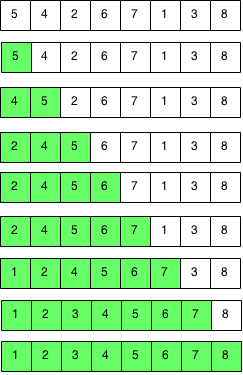## Introduction

Insertion is a sort that works by inserting unused elements into a sorted array. We start with an empty array and we add the first element to the array. We then add the second element to the array and we "insert" it by shifting elements. We keep doing this until the array is sorted.

## Implementation

We split the original array into two parts: the first part is sorted and the second part is unsorted. We take the first element from the second part and insert it into the sorted part. We keep doing this until we have no more elements in the unsorted part and we only have the sorted part.### Code

```public static void insertionSort(int[] array) {
int i,j;

// Iterate through size of array.
for (j = 1; j < array.length; j++) {
int element = array[j];
// Shift all elements until beginning of array or correct position.
for (i = j - 1; (i >= 0) && (array[i] < element); i--) {
array[i + 1] = array[i];
}
// Insert element into correct position.
array[i + 1] = element;
}
}
```